## Filters

Sort by :
Q
Are there any other states of matter exist other than Solid, Liquid and Gasses?
Yes. There are five known Phases or States of the matter: solids, liquids, gases, plasma, and Bose-Einstein condensates. You will study these in higher classes.

10 identical cell in series are connected to the ends of a resistance of 59,the current is  found to be 0.25A. But when the same cells being connected in parallel,are joined to the ends of a resistance of 0.05,the current is 25A EMF of  each cell is

A.

1V

B.

0.5V

C.

1.5V

D.

2V

In series:  In parallel:  (1)-(2)

Question

Two finite sets have m and n elements. The total number  subsets of first set is 56 more than second set, find m and n.

A.B.C.D.Magnetic field through a coil is changed with respect to time then emf induced in it then select the incorrect regarding induced emf in coil

Option (1) is Incorrect as the coil must be made of conducting substance if we want to induce emf and pass current through it.
1560259998516566813835.jpg@Vinod

View More

Letandbe the roots of equationis equal to:

• Option 1)

6

• Option 2)

-6

• Option 3)

3

• Option 4)

-3

3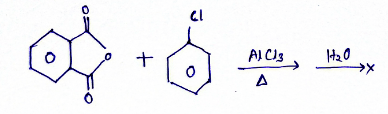Compound X will be

• Option 1)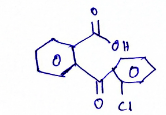• Option 2)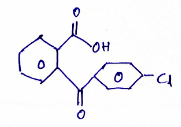• Option 3)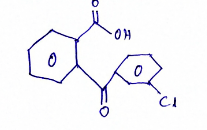• Option 4)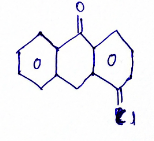2

Find the compound "X" which give the following test:

Neutral FeCl3  -Ve

Fehling Solution  -ve

Iodoform reaction  +ve

Grignard reagent  +ve

• Option 1)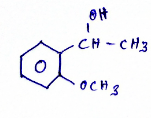• Option 2)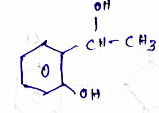• Option 3)